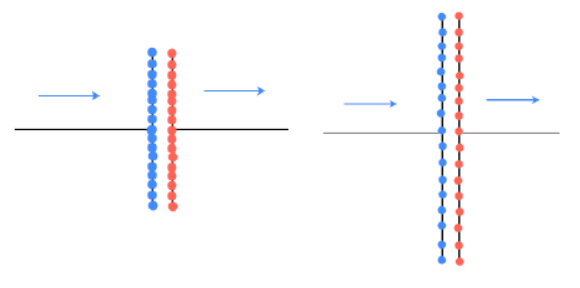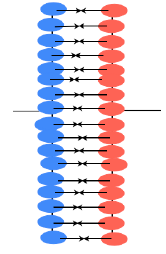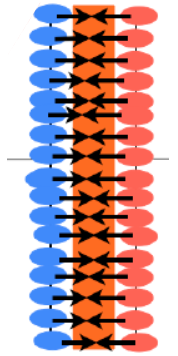### Area of the capacitor platesThe larger the area of the plates the more spread out the charge becomes and more charge can be pushed onto the plates.

The larger the area of the plates the larger the capacitor because there is more space to store charge. The size of the capacitor is directly proportional to the size of the plates, so:

CαA

### Capacitance and plate spacingThe charges on opposite plates attract one another. The nearer together the plates then the stronger that attraction is and the greater the amount of charge the plates will hold.

The size of the capacitor is then inversely proportional to the distance between the plates, so:

C α 1/d

### Capacitance and dielectric

The dielectric is the material between the plates. It does three things:It insulates one plate from the other so the charges do not cancel each other out. Because the plates are very close together the dielectric must be a very good insulator.

The dielectric keeps the plates apart. If the capacitor is “rolled up” then the plates are squashed together, but they must not touch.

The dielectric can make the electric field between the plates stronger. That means that the charges attract each other more and therefore more charge can be held on the plates. The ability of the dielectric to support an electric field is called the permittivity. So the size of the capacitance is directly proportional to the permittivity, ε, so:

ε

In fact air is quite a good dielectric and it is cheap and so it is sometimes used but it cannot be used if the charged plates or foils are rolled up. A vacuum is also quite good, but that is rather impractical. The most common dielectrics are plastic films and oxide layers. We often compare the dielectrics with a vacuum so that their value of permittivity is written like this: ε = kε0 so k is a constant for that material called the relative permittivity.

### The overall equation

Putting all of these connections together we get:

C= ε A/d where A is area ε is permittivity of the dielectric and d is the spacing between the plates.

This can sometimes be given as:

C= kε0 A/d  whereε0 is the permittivity of free space and k is the relative permittivity of the material